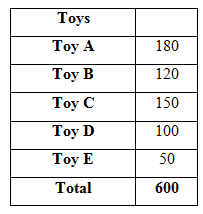# SBI Clerk Prelims 2021 Quantitative Aptitude Questions (Day-03)

Dear Aspirants, Our IBPS Guide team is providing new series of Quantitative Aptitude Questions for SBI Clerk Prelims 2020 so the aspirants can practice it on a daily basis. These questions are framed by our skilled experts after understanding your needs thoroughly. Aspirants can practice these new series questions daily to familiarize with the exact exam pattern and make your preparation effective.

Start Quiz

Directions (1-5): What approximate value should come in the place of (?) in the following questions?

1) 16.10 * 22.12 ÷ ? + 250 = 315.12

A.5

B.10

C.15

D.25

E.30

2) 110% of 520.1809 + 10.12% of 1584.72 = ?

A.451

B.651

C.731

D.891

E.961

3) 10.12 * 30.8 * ? = 3512.10 + 48.14

A.12

B.24

C.36

D.48

E.52

4) 359.99 * 14.10 ÷ 3.002 + 24.88 = ?

A.1390

B.1405

C.1705

D.1910

E.2345

5) 8259.998 ÷ 40.001 = ? – 512.998

A.128

B.450

C.490

D.720

E.870

Directions (6-8): Study the following information carefully and answer the questions given below.

A manufacturing company produced five different types of toys. viz., Toy A, Toy B, Toy C, Toy D and Toy E. Total number of all the five toys is 600. 30% of the total number of toys is toy A. One fifth of the total number of toys is toy B. 25% of the total number of toys is toy C. Remaining toys are either toy D or toy E. Number of toy E is 50 less than the number of toys D produced.

6) Total number of toys D produced is approximately what percent of the total number of toys C and toys B produced together?

A.37%

B.45%

C.56%

D.78%

E.81%

7) What is the total number of toys A, toys C and toys E produced by the company?

A.250

B.380

C.120

D.780

E.None of these

8) What is the ratio of the total number of toys C and toys E produced to the total number of toys A and toys B produced?

A.2: 3

B.3: 4

C.2: 7

D.7: 9

E.None of these

Directions (9-10) : In each of the following questions, two equations are given. You have to solve both the equations to find the relation between x and y.

9) I: 3x2+2x – 1 = 0

II: 11y2 + 18y + 7 = 0

A.If x < y

B.If x > y

C.If x ≤ y

D.If x ≥ y

E.If relationship between x and y cannot be determined

10) I: 6x2 + 19x – 7 = 0

II: y2-11y + 24 = 0

A.If x < y

B.If x > y

C.If x ≤ y

D.If x ≥ y

E.If relationship between x and y cannot be determined

Directions (1-5) :

16.10 * 22.12 ÷ ? + 250 = 315.12

=> 16 * 22 ÷ ? + 250 = 315

=> 352/? = 65

=> 5

110% of 520.1809 + 10.12% of 1584.72 = ?

=> 110% of 520 + 10% of 1585 = ?

=> 572 + 159 = ?

=> 731

10.12 * 30.8 * ? = 3512.10 + 48.14

=> 10 * 31 * ? = 3512 + 48

=> 12

359.99 * 14.10 ÷ 3.002 + 24.88 = ?

=> 360 * (14/3) + 25 = ?

=> 120 * 14 + 25 = ?

=> 1705

8259.998 ÷ 40.001 = ? – 512.998

=> 8260 ÷ 40 = ? – 513

=> 207 + 513 = ?

=> 720

Directions (6-8) :

Total number of toys = 600

Toy A = 30% of 600 = 180

Toy B = 1/5 * 600 = 120

Toy C = 25% of 600 = 150

Remaining toys = Toy D + Toy E = 600 – 180 – 120 – 150 = 150

Toy D + Toy E = 150 and Toy D – Toy E = 50

Hence, Toy D = 100 and Toy E = 50Toys D = 100

Toys C + Toys B = 150 + 120 = 270

Required % = 100/270 * 100 ≈ 37%

Toys A + Toys C + Toys E = 180 + 150 + 50 = 380

Toys C + Toys E = 150 + 50 = 200

Toys A + Toys B = 180 + 120 = 300

Required ratio = 200: 300 = 2: 3

Directions (9-10) :

From I =>3x2 + 2x – 1 = 0

=> 3x(x + 1) – (x + 1) = 0

=> x = 1/3, -1

From II =>11y2 + 18y + 7 = 0

=> 11y(y + 1) + 7(y + 1) = 0

=> y = -7/11, -1

Hence, relationship between x and y cannot be determined.

From I =>6x2 + 19x – 7 = 0

=> (3x – 1) (2x + 7) = 0

=> x = 1/3, -7/2

From II =>y2 – 11y + 24 = 0

=>y(y – 3) – 8(y – 3) = 0

=>y = 3, 8

Hence, x < y